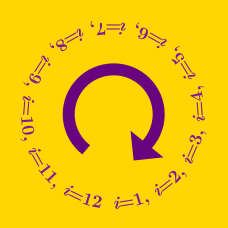Computer Science

# Loops: Level 3 Challenges

Find the sum of all primes less than 1 million such that they are 1 more than a perfect square.

Note-As an explicit example, 2- which is 1 greater than 1 (a perfect square) is a prime and thus satisfies the above condition.

### Inspired by Vighnesh Raut's "Prime sum"$\sqrt{ \text{PASSION} } = \text{KISS}$

If each letter represents a different digit from 0 to 9, what is the value of KISS?

A HOTEL with 1000 rooms has 1000 waiters. Each waiter and room is given a number from 1-1000.

Initially, waiter with number 1 goes and closes doors of all rooms.
After that waiter with number 2 goes and changes the state of doors corresponding to multiples of that 2.
After that waiter number 3 goes and changes the state of doors corresponding to multiples of 3.
So on and so forth

Find the number of doors that are open after the 1000th waiter has returned .

NOTE: Changes the state of door means that opened doors are closed and closed doors are opened.

The function $\pi(x)$ gives the number of primes less or equal to $x$. Find the first three digits of $\pi( 2 \times 10^{11} )$.

Define $\text{sum}(N)$ which returns the sum of digits of a number.

Find the smallest pair of consecutive primes $a$ and $b$ which such that $\text{sum}(a) = \text{sum}(b)$. Enter your answer as $a + b$.

Details and Assumptions:

• Consecutive prime numbers refers to a sequence of two prime numbers which don't have any prime number between them. For example: 2 and 3 are consecutive primes, 37 and 41 are consecutive primes.

• As an explicit example: $\text{sum}(37) \neq \text{sum}(41)$ because $3+7\neq 4+1$. So 37 and 41 are not such numbers.

×

Problem Loading...

Note Loading...

Set Loading...